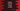# Python program to convert a string to a sequence of byte## Python program to convert a string to a sequence of bytes:

Converting a string to a sequence of bytes is called encoding. A sequence of bytes or a byte object can be stored directly on the disk. We can’t directly store a string on disk. For that, we need to convert a string to a sequence of bytes or we need to encode the string.

### Method 1: Using bytes() method:

bytes is an inbuilt method in python and we can use it to convert a string to byte array.

This method is defined as below:

``bytes([src[,encoding[,err]]])``

Here,

• all the three parameters are optional.
• src is the source we are using to convert to a byte object. In this example, it is a string.
• encoding is the encoding that we want to use with the source.
• err is the action to perform if the encoding fails.

### Example of string to bytes array:

Let’s take a look at the below program:

``````given_string = 'Hello'

arr_utf_8 = bytes(given_string, 'utf-8')
arr_utf_16 = bytes(given_string, 'utf-16')
arr_ascii = bytes(given_string, 'ascii')

print('utf-8: ')
for byte in arr_utf_8:
print(byte, end=' ')
print()

print('utf-16: ')
for byte in arr_utf_16:
print(byte, end=' ')
print()

print('ascii: ')
for byte in arr_ascii:
print(byte, end=' ')
print()``````

Here,

• we used utf-8, utf-16 and ascii encodings for the same string.

If you run this program, it will print the below output:

``````utf-8:
72 101 108 108 111
utf-16:
255 254 72 0 101 0 108 0 108 0 111 0
ascii:
72 101 108 108 111 ``````

### Method 2: Using string.encode:

Python string comes with a method to encode it to a byte object. This is defined as below:

``str.encode(encoding, err)``

Here,

• encoding is the encoding to use. By default it is utf-8
• err is a error handling scheme. It is strict by default.

Let’s change the above program to use string.encode:

``````given_string = 'Hello'

arr_utf_8 = given_string.encode('utf-8')
arr_utf_16 = given_string.encode('utf-16')
arr_ascii = given_string.encode('ascii')

print('utf-8: ')
for byte in arr_utf_8:
print(byte, end=' ')
print()

print('utf-16: ')
for byte in arr_utf_16:
print(byte, end=' ')
print()

print('ascii: ')
for byte in arr_ascii:
print(byte, end=' ')
print()``````

It will print the same output.